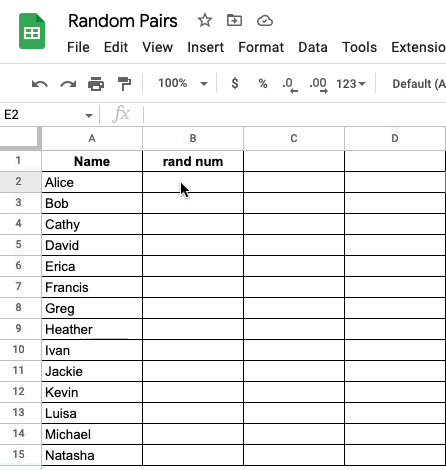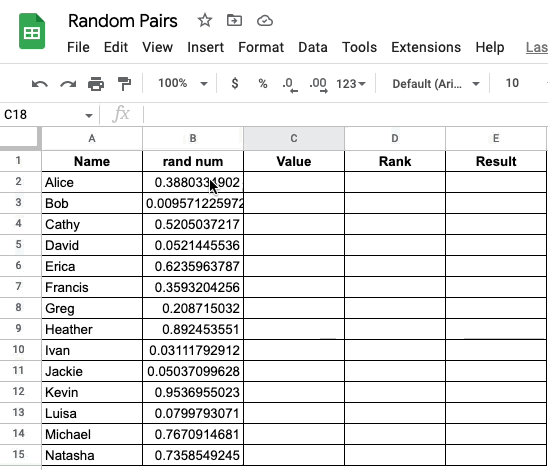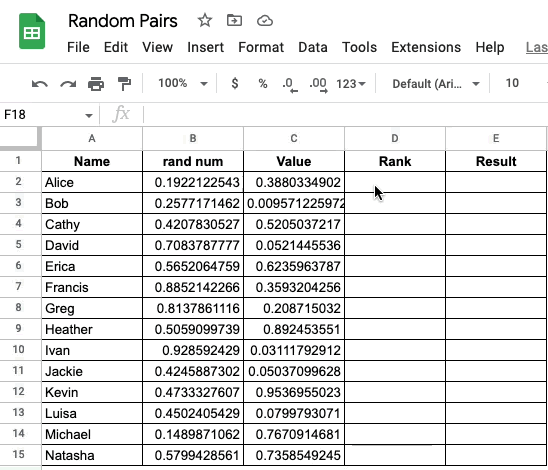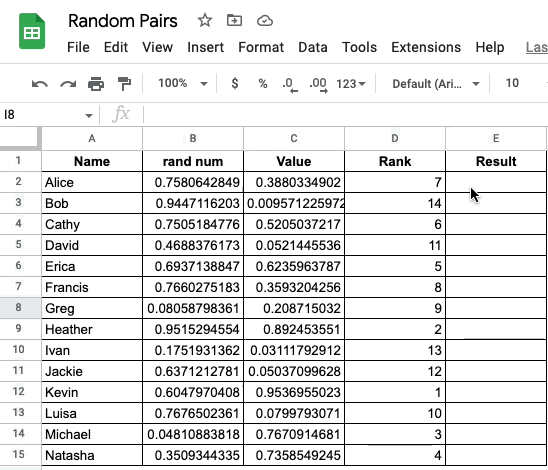This week, I got this task to randomly generate pairs based on a name list. After googling on the internet, I found a perfect solution to achieve this.

Here are the detailed steps to achieve a random pair of two. All the example data and formula can be found in this Google Sheet Random Pairs .

## Step 1 - Generate random numbers using `RAND()`### Step 2 - Copy the random number values to a new column named Value#

Excel or Google Sheets will automatic re-generate a new series of random numbers with the `RAND()` function. An easy solution is to just copy these random values as a new column.## Step 3 - Create ranking on column Value with `RANK()`#

When we use the `RANK()` function, one thing needs to be careful is to put the `\$` sign to lock the data section that you will use in the `RANK()` function.## Step 4 - Pair up people with `CEILING()`#

Here we use a formula combination of `TEXTJOIN()` and `ceiling()` to create the random pair.

If you need to create a random pair of three, you just need to change the formula to something like `=TEXTJOIN(" ", TRUE, D2, ceiling(D2/2)`.Hope it helps.